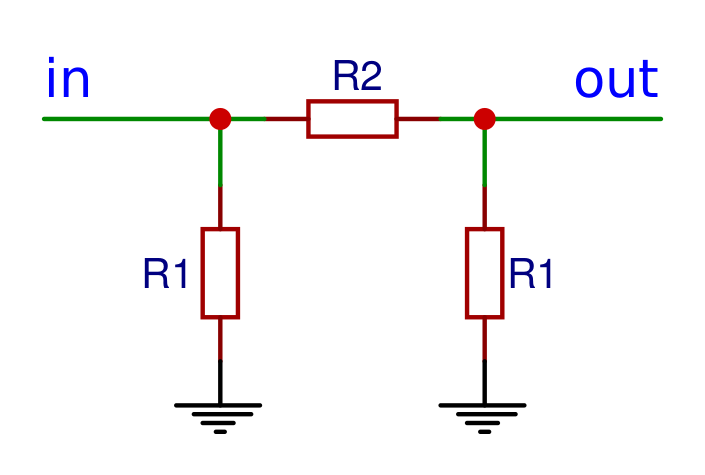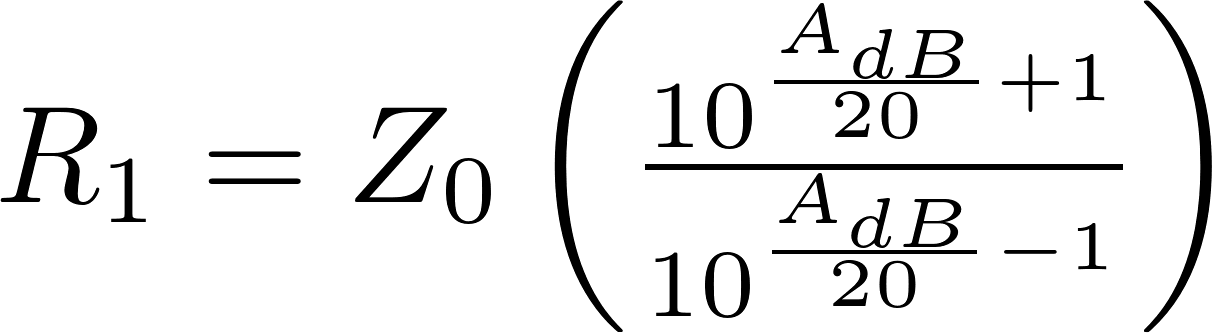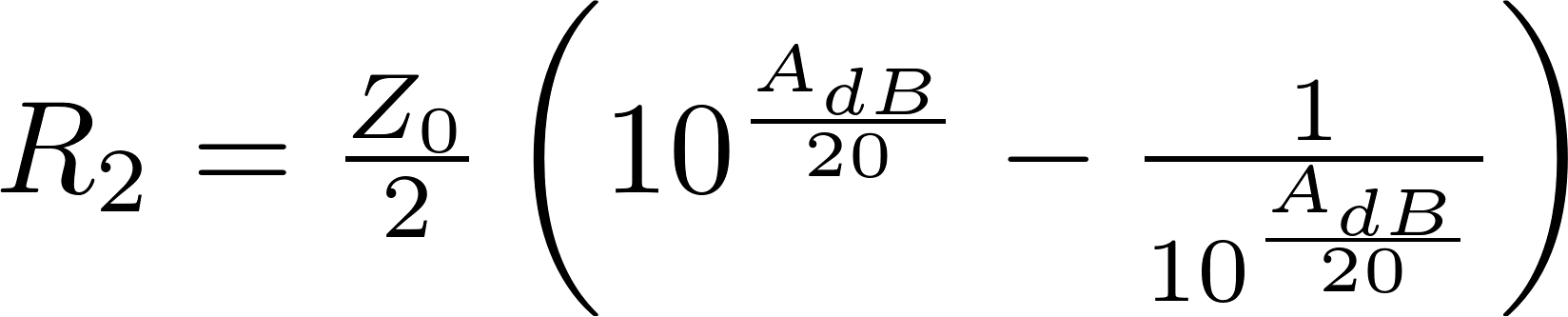# Pi attenuator

The Pi attenuator is a type of attenuator that looks like the Greek letter π. The Pi attenuator consists of three resistors. One of these is connected in series and the other two are connected in parallel to ground. The parallel resistors often have the same resistance.

### Formulas

The following formulas can be used to calculate the resistance of all resistors for a given attenuation and impedance.### Calculator

Enter the attenuation and impedance to calculate the resistance of the resistors.